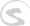Documents
Writing Docs Gigedit SFZ Instrument Scripts NKSP Language NKSP Reference

# abs()

Returns the absolute value of the passed value argument. That means, if a positive number or zero is passed, then the same number is returned. If however a negative number is passed, then the signment of the number is flipped to positive. So it behaves like simply removing the minus sign if any.

### Function Prototype

`abs(number) `

### Arguments

Argument Name Data Type Description
`number` Integer Number or
Real Number
The number to be converted.
[required]

### Return Value

Data Type Description
Same as `number` Absolute value of the passed number.

### Remarks

This functions accepts any standard unit and finalness for its argument `number`. The return value's standard unit and finalness is identical to the passed `number`'s one.

### Examples

• on init
• declare %values := ( -3, -2, -1, 0, 1, 2, 3 )
• declare \$i := 0
• while (\$i < num_elements(values))
• message("The absolute value of " & \$values[\$i] & " is " & abs(\$values[\$i]))
• end while
• end on
Document Updated:  2019-09-16  |  Author:  Christian Schoenebeck